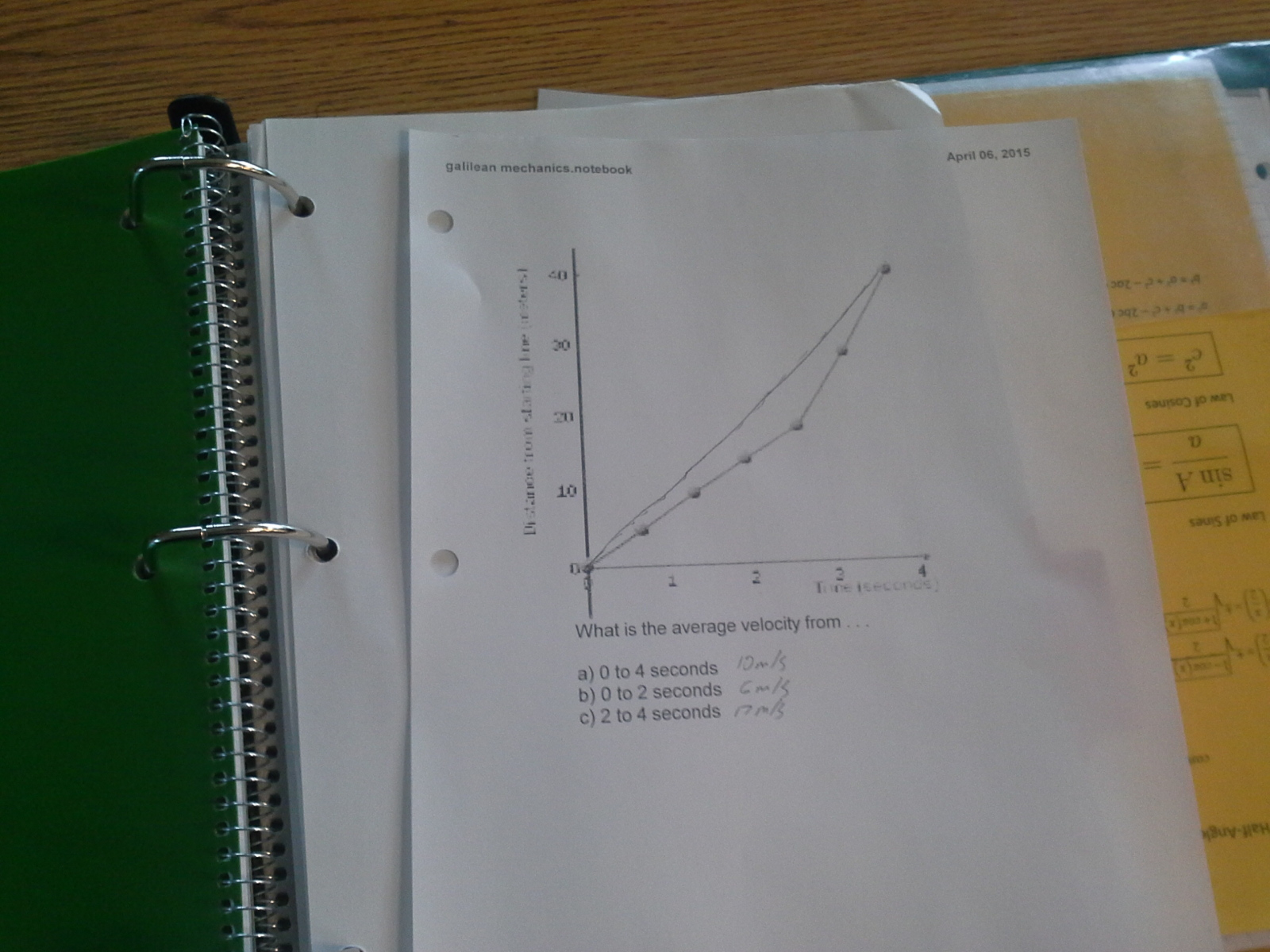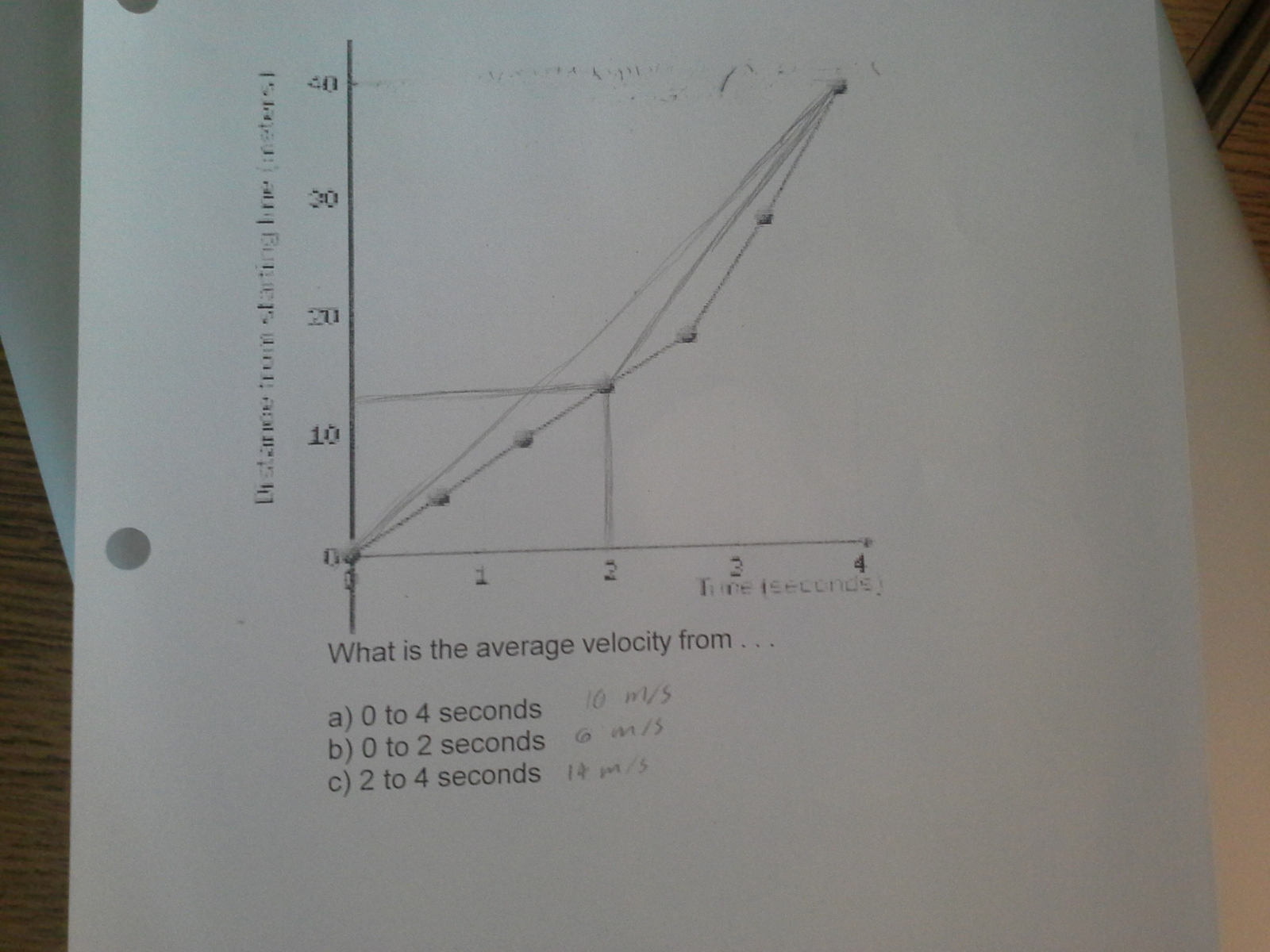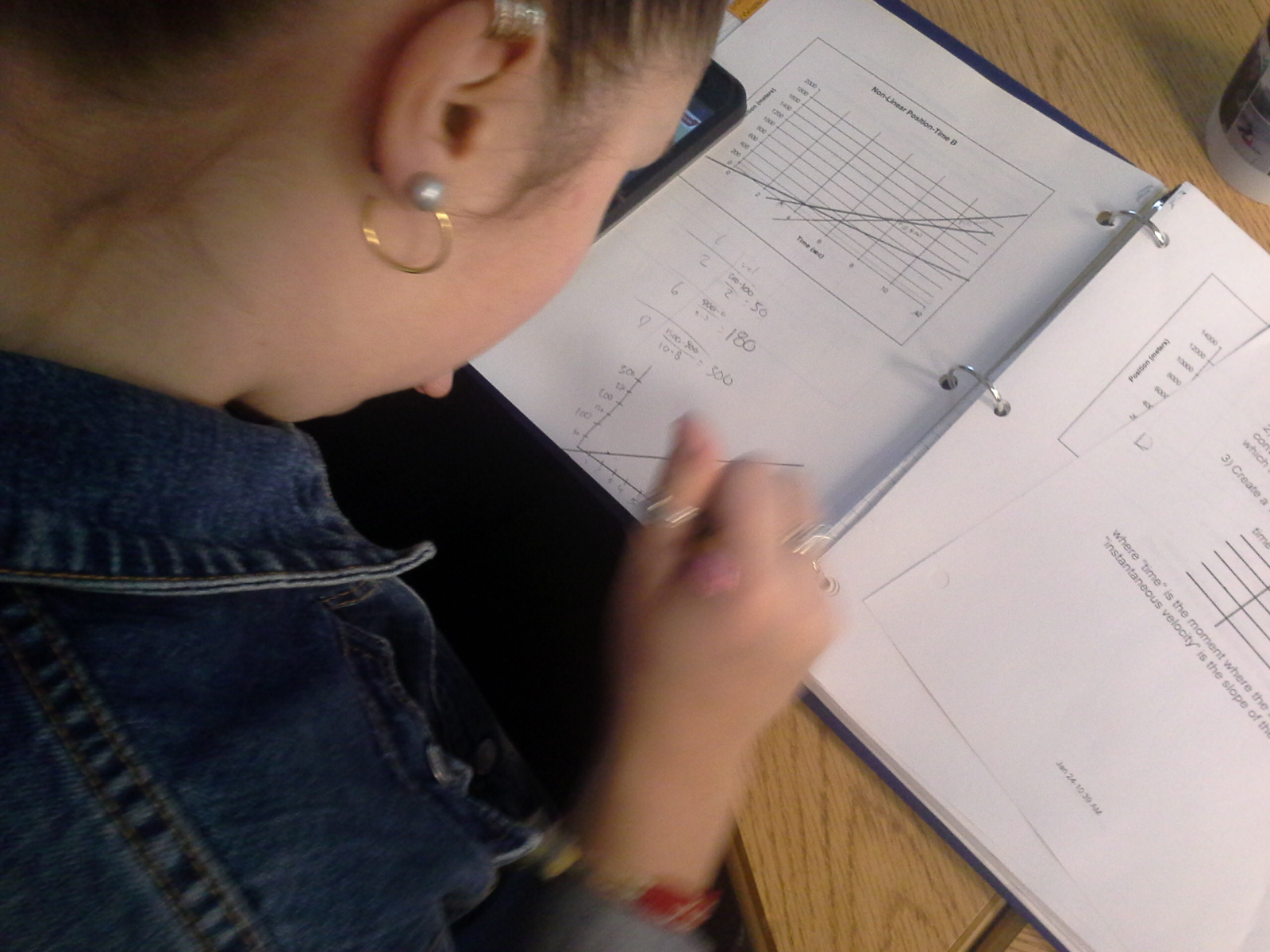# Nonlinear Position Graphs

## Objective

Students will develop a technique for analyzing nonlinear position graphs to produce velocity graphs.

#### Big Idea

Tangent lines are used to determine instantaneous velocities.

## Warmup

15 minutes

We continue our study of objects in motion with a lesson focusing on nonlinear position graphs. The student's mantra ("The slope of a position versus time graph is the velocity") is stretched to cover the case of "instantaneous velocities." By the end of the lesson, students should be able to determine the acceleration of an object whose velocity is not constant. This is an important step toward the demonstration of NGSS Performance Expectation HS-PS2-1.

I begin the day with a warmup that looks familiar - position graphs that feature linear segments- but has a small twist: I ask for an average velocity in three regions of the function, two of which cross over the segments. Students quite naturally, and correctly, take the position and time information for each region and compute a velocity in the same way that one would compute a slope. After about five minutes or so, I show solutions at the board and incorporate a critical idea: the definition of velocity depends only on the end points being considered; the path is irrelevant.

Here, a student shows the connection of the end points for the first part of the warmup. the slope of this segment is the average velocity over the interval under consideration (0 to 4 seconds).A fuller solution is shown below. Straight line segments identify each of the three intervals and the associated slopes are recorded. These slopes are the average velocities for the intervals. The student handout is, unfortunately, fainter than the original which leads students to estimate the position at two seconds to be 12 meters. This explains the discrepancy between these solutions and the one provided above. Nevertheless, the concept is clearly demonstrated.## Nonlinear Position Graphs Lecture

30 minutes

I begin this lecture portion of the class by sharing the overall goal: to create a reasonable velocity graph from a nonlinear position graph. This is exactly the same problem as the one students wrestled with in the previous class, so building upon their ideas is a natural progression.

I solicit student ideas about their first thoughts about a nonlinear position graph. Many students want to "straighten" the curve by imposing a line over small portions of the curve. I add in a line like that and ask students to mentally zoom in to see that a straight line will always cut across the curve. A good idea is to approximate an interval with a straight line. A better idea is to make the interval smaller. The best idea is to make the interval essentially zero - the slope of a line that touches the curve at one point, or a tangent line. This kind of line will produce an "instantaneous velocity" and, if we continue the process for several tangent lines, we can create a T-chart of times and velocities.

At this point, I ask students to draw in 4-5 tangent lines on this practice problem and find their slopes. While they do so, I hand out a set of notes where the first part captures what we've done so far and the second part shows the next steps.

Students contribute their data to the class board and I take this data and demonstrate the next part of the process - making a velocity versus time graph that is scaled with some "modest care." I show the construction of a graph, plot a few points, then eyeball a best fit line.

This estimated velocity versus time function has a slope and a y-intercept like any straight line. Both of these features have important physical meanings: the slope is the acceleration and the y-intercept is the initial velocity. Together, we write the linear function that describes this velocity versus time graph. I then give students some time to practice the three other three problems in the next segment of class.

## Practice with Nonlinear Position Graphs

30 minutes

Students begin a practice session with the other three practice problems on the handout (the fourth problem is the example problem from earlier today). They may work individually or collaborate, though each student should create his or her own set of tangent lines and corresponding slopes. I circulate around the room, checking in with students to see their progress or their struggles.

As students finish a problem, they add their acceleration and initial velocity estimates to the table on the board. In this way, we can provide some affirmation for those who are working more slowly, while also noting the inevitable variation that this process produces. Students continue with this practice until there are about five minutes left in class.

Here is an excellent example of a solution to problem c:And here a student works her way through problem b - note the hand-made scale she has created for the velocity graph. Students are encouraged to make these velocity graphs quickly though with an attempt to keep a reasonable scale to their axes. While this obviously leads to differences in student estimates of coordinate values and the slopes that are based on those values, we can gather multiple student responses and average them to get satisfying final answers. In this way, I can promote a spirit of "back-of-the-envelope" estimation with my students while preserving a sense of accuracy to the results. We can also work quickly; measuring out scales and plotting points more precisely would take time and I want to get more practice with the concept linking velocities to slopes of tangent lines.## Exit Ticket

10 minutes

In the final few minutes, I ask students to quickly sketch three more velocity graphs in their notebooks. I show the prompt on the board and am hopeful that they can decode the information immediately. The acceleration and the initial velocity is provided for each problem, either directly or encoded as a slope and y-intercept of a straight line velocity function. What I'm probing for is their understanding of the final piece of this long process: that slopes and y-intercepts of velocity functions have a physical meaning.

The very last question - the generation of a position graph from some velocity information - requires a bit more time and energy. I put it out there for students to consider the mental agility needed to accomplish this task; it requires a reversal of today's thinking.